# Bitmap example: Animated spinning moon

Flash Player 9 and later, Adobe AIR 1.0 and later

The Animated spinning moon example demonstrates techniques for working with Bitmap objects and bitmap image data (BitmapData objects). The example creates an animation of a spinning, spherical moon using a flat image of the moon’s surface as the raw image data. The following techniques are demonstrated:

• Creating animation by repeatedly copying pixels from different parts of a source image

• Creating a bitmap image by setting pixel values

To get the application files for this sample, see www.adobe.com/go/learn_programmingAS3samples_flash . The Animated spinning moon application files can be found in the Samples/SpinningMoon folder. The application consists of the following files:

File

Description

SpinningMoon.mxml

or

SpinningMoon.fla

The main application file in Flex (MXML) or Flash (FLA).

com/example/programmingas3/moon/MoonSphere.as

Class that performs the functionality of loading, displaying, and animating the moon.

moonMap.png

Image file containing a photograph of the moon’s surface, which is loaded and used to create the animated, spinning moon.

The first main task this sample performs is loading an external image file, which is a photograph of the moon’s surface. The loading operation is handled by two methods in the MoonSphere class: the MoonSphere() constructor, where the loading process is initiated, and the imageLoadComplete() method, which is called when the external image is completely loaded.

```var imageLoader:Loader = new Loader();

```private function imageLoadComplete(event:Event):void
{
textureMap = event.target.content.bitmapData;
...
}```

## Creating animation by copying pixels

A basic definition of animation is the illusion of motion, or change, created by changing an image over time. In this sample, the goal is to create the illusion of a spherical moon rotating around its vertical axis. However, for the purposes of the animation, you can ignore the spherical distortion aspect of the sample. Consider the actual image that’s loaded and used as the source of the moon image data:

View full size graphic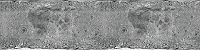As you can see, the image is not one or several spheres; it’s a rectangular photograph of the surface of the moon. Because the photo was taken exactly at the moon’s equator, the parts of the image that are closer to the top and bottom of the image are stretched and distorted. To remove the distortion from the image and make it appear spherical, we will use a displacement map filter, as described later. However, because this source image is a rectangle, to create the illusion that the sphere is rotating, the code simply needs to slide the moon surface photo horizontally.

Notice that the image actually contains two copies of the moon surface photograph next to each other. This image is the source image from which image data is copied repeatedly to create the appearance of motion. By having two copies of the image next to each other, a continuous, uninterrupted scrolling effect can more easily be created. Let’s walk through the process of the animation step-by-step to see how this works.

The process actually involves two separate ActionScript objects. First, there is the loaded source image, which in the code is represented by the BitmapData instance named textureMap . As described previously, textureMap is populated with image data as soon as the external image loads, using this code:

`textureMap = event.target.content.bitmapData;`

The content of textureMap is the rectangle moon image. In addition, to create the animated rotation, the code uses a Bitmap instance named sphere , which is the actual display object that shows the moon image onscreen. Like textureMap , the sphere object is created and populated with its initial image data in the imageLoadComplete() method, using the following code:

```sphere = new Bitmap();
sphere.bitmapData = new BitmapData(textureMap.width / 2, textureMap.height);
sphere.bitmapData.copyPixels(textureMap,
new Rectangle(0, 0, sphere.width, sphere.height),
new Point(0, 0));```

As the code shows, sphere is instantiated. Its bitmapData property (the raw image data that is displayed by sphere ) is created with the same height and half the width of textureMap . In other words, the content of sphere will be the size of one moon photo (since the textureMap image contains two moon photos side-by-side). Next the bitmapData property is filled with image data using its copyPixels() method. The parameters in the copyPixels() method call indicate several things:

• The first parameter indicates that the image data is copied from textureMap .

• The second parameter, a new Rectangle instance, specifies from which part of textureMap the image snapshot should be taken; in this case the snapshot is a rectangle starting from the top left corner of textureMap (indicated by the first two Rectangle() parameters: 0, 0 ) and the rectangle snapshot’s width and height match the width and height properties of sphere .

• The third parameter, a new Point instance with x and y values of 0 , defines the destination of the pixel data—in this case, the top-left corner (0, 0) of sphere.bitmapData .

Represented visually, the code copies the pixels from textureMap outlined in the following image and pastes them onto sphere . In other words, the BitmapData content of sphere is the portion of textureMap highlighted here:

View full size graphic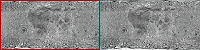Remember, however, that this is just the initial state of sphere —the first image content that’s copied onto sphere .

With the source image loaded and sphere created, the final task performed by the imageLoadComplete() method is to set up the animation. The animation is driven by a Timer instance named rotationTimer , which is created and started by the following code:

```var rotationTimer:Timer = new Timer(15);
rotationTimer.start();```

The code first creates the Timer instance named rotationTimer ; the parameter passed to the Timer() constructor indicates that rotationTimer should trigger its timer event every 15 milliseconds. Next, the addEventListener() method is called, specifying that when the timer event ( TimerEvent.TIMER ) occurs, the method rotateMoon() is called. Finally, the timer is actually started by calling its start() method.

Because of the way rotationTimer is defined, approximately every 15 milliseconds Flash Player calls the rotateMoon() method in the MoonSphere class, which is where the animation of the moon happens. The source code of the rotateMoon() method is as follows:

```private function rotateMoon(event:TimerEvent):void
{
sourceX += 1;
if (sourceX > textureMap.width / 2)
{
sourceX = 0;
}

sphere.Data.copyPixels(textureMap,
new Rectangle(sourceX, 0, sphere.width, sphere.height),
new Point(0, 0));

event.updateAfterEvent();
}```

The code does three things:

1. The value of the variable sourceX (initially set to 0) increments by 1.

`sourceX += 1;`

As you’ll see, sourceX is used to determine the location in textureMap from which the pixels will be copied onto sphere , so this code has the effect of moving the rectangle one pixel to the right on textureMap . Going back to the visual representation, after several cycles of animation the source rectangle will have moved several pixels to the right, like this:

View full size graphic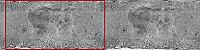After several more cycles, the rectangle will have moved even farther:

View full size graphic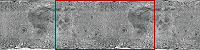This gradual, steady shift in the location from which the pixels are copied is the key to the animation. By slowly and continuously moving the source location to the right, the image that is displayed on the screen in sphere appears to continuously slide to the left. This is the reason why the source image ( textureMap ) needs to have two copies of the moon surface photo. Because the rectangle is continually moving to the right, most of the time it is not over one single moon photo but rather overlaps the two moon photos.

2. With the source rectangle slowly moving to the right, there is one problem. Eventually the rectangle will reach the right edge of textureMap and it will run out of moon photo pixels to copy onto sphere :

View full size graphic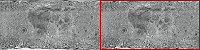The next lines of code address this issue:

```if (sourceX >= textureMap.width / 2)
{
sourceX = 0;
}```

The code checks if sourceX (the left edge of the rectangle) has reached the middle of textureMap . If so, it resets sourceX back to 0, moving it back to the left edge of textureMap and starting the cycle over again:

View full size graphic3. With the appropriate sourceX value calculated, the final step in creating the animation is to actually copy the new source rectangle pixels onto sphere . The code that does this is very similar to the code that initially populated sphere (described previously); the only difference is that in this case, in the new Rectangle() constructor call, the left edge of the rectangle is placed at sourceX :

```sphere.bitmapData.copyPixels(textureMap,
new Rectangle(sourceX, 0, sphere.width, sphere.height),
new Point(0, 0));```

Remember that this code is called repeatedly, every 15 milliseconds. As the source rectangle’s location is continuously shifted, and the pixels are copied onto sphere , the appearance on the screen is that the moon photo image represented by sphere continuously slides. In other words, the moon appears to rotate continuously.

## Creating the spherical appearance

The moon, of course, is a sphere and not a rectangle. Consequently, the sample needs to take the rectangular moon surface photo, as it continuously animates, and convert it into a sphere. This involves two separate steps: a mask is used to hide all the content except for a circular region of the moon surface photo, and a displacement map filter is used to distort the appearance of the moon photo to make it look three-dimensional.

First, a circle-shaped mask is used to hide all the content of the MoonSphere object except for the sphere created by the filter. The following code creates the mask as a Shape instance and applies it as the mask of the MoonSphere instance:

```moonMask = new Shape();

Note that since MoonSphere is a display object (it is based on the Sprite class), the mask can be applied directly to the MoonSphere instance using its inherited mask property.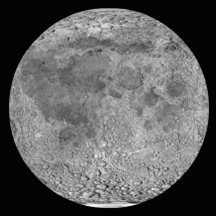Simply hiding parts of the photo using a circle-shaped mask isn’t enough to create a realistic-looking rotating-sphere effect. Because of the way the photo of the moon’s surface was taken, its dimensions aren’t proportional; the portions of the image that are more toward the top or bottom of the image are more distorted and stretched compared to the portions in the equator. To distort the appearance of the moon photo to make it look three-dimensional, we’ll use a displacement map filter.

A displacement map filter is a type of filter that is used to distort an image. In this case, the moon photo will be “distorted” to make it look more realistic, by squeezing the top and bottom of the image horizontally, while leaving the middle unchanged. Assuming the filter operates on a square-shaped portion of the photo, squeezing the top and bottom but not the middle will turn the square into a circle. A side effect of animating this distorted image is that the middle of the image seems to move farther in actual pixel distance than the areas close to the top and bottom, which creates the illusion that the circle is actually a three-dimensional object (a sphere).

The following code is used to create the displacement map filter, named displaceFilter :

```var displaceFilter:DisplacementMapFilter;
displaceFilter = new DisplacementMapFilter(fisheyeLens,
BitmapDataChannel.RED,
BitmapDataChannel.GREEN,

The first parameter, fisheyeLens , is known as the map image; in this case it is a BitmapData object that is created programmatically. The creation of that image is described in Creating a bitmap image by setting pixel values . The other parameters describe the position in the filtered image at which the filter should be applied, which color channels will be used to control the displacement effect, and to what extent they will affect the displacement. Once the displacement map filter is created, it is applied to sphere , still within the imageLoadComplete() method:

`sphere.filters = [displaceFilter];`

The final image, with mask and displacement map filter applied, looks like this: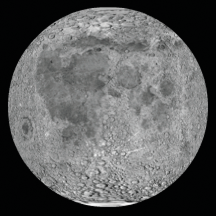With every cycle of the rotating moon animation, the BitmapData content of sphere is overwritten by a new snapshot of the source image data. However, the filter does not need to be re-applied each time. This is because the filter is applied to the Bitmap instance (the display object) rather than to the bitmap data (the raw pixel information). Remember, the Bitmap instance is not the actual bitmap data; it is a display object that displays the bitmap data on the screen. To use an analogy, a Bitmap instance is like the slide projector that is used to display photographic slides on a screen, and a BitmapData object is like the actual photographic slide that can be presented through a slide projector. A filter can be applied directly to a BitmapData object, which would be comparable to drawing directly onto a photographic slide to alter the image. A filter can also be applied to any display object, including a Bitmap instance; this would be like placing a filter in front of the slide projector’s lens to distort the output shown on the screen (without altering the original slide at all). Because the raw bitmap data is accessible through a Bitmap instance’s bitmapData property, the filter could have been applied directly to the raw bitmap data. However, in this case, it makes sense to apply the filter to the Bitmap display object rather than to the bitmap data.

For detailed information about using the displacement map filter in ActionScript, see Filtering display objects .

## Creating a bitmap image by setting pixel values

One important aspect of a displacement map filter is that it actually involves two images. One image, the source image, is the image that is actually altered by the filter. In this sample, the source image is the Bitmap instance named sphere . The other image used by the filter is known as the map image. The map image is not actually displayed on the screen. Instead, the color of each of its pixels is used as an input to the displacement function—the color of the pixel at a certain x, y coordinate in the map image determines how much displacement (physical shift in position) is applied to the pixel at that x, y coordinate in the source image.

Consequently, to use the displacement map filter to create a sphere effect, the sample needs the appropriate map image—one that has a gray background and a circle that’s filled with a gradient of a single color (red) going horizontally from dark to light, as shown here: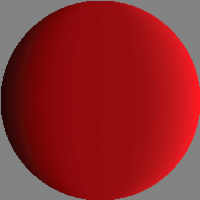Because only one map image and filter are used in this sample, the map image is only created once, in the imageLoadComplete() method (in other words, when the external image finishes loading). The map image, named fisheyeLens , is created by calling the MoonSphere class’s createFisheyeMap() method:

`var fisheyeLens:BitmapData = createFisheyeMap(radius);`

Inside the createFisheyeMap() method, the map image is actually drawn one pixel at a time using the BitmapData class’s setPixel() method. The complete code for the createFisheyeMap() method is listed here, followed by a step-by-step discussion of how it works:

```private function createFisheyeMap(radius:int):BitmapData
{
var diameter:int = 2 * radius;

var result:BitmapData = new BitmapData(diameter,
diameter,
false,
0x808080);

// Loop through the pixels in the image one by one
for (var i:int = 0; i < diameter; i++)
{
for (var j:int = 0; j < diameter; j++)
{
// Calculate the x and y distances of this pixel from
// the center of the circle (as a percentage of the radius).

// Calculate the linear distance of this pixel from
// the center of the circle (as a percentage of the radius).
var pctDistance:Number = Math.sqrt(pctX * pctX + pctY * pctY);

// If the current pixel is inside the circle,
// set its color.
if (pctDistance < 1)
{
// Calculate the appropriate color depending on the
// distance of this pixel from the center of the circle.
var red:int;
var green:int;
var blue:int;
var rgb:uint;
red = 128 * (1 + 0.75 * pctX * pctX * pctX / (1 - pctY * pctY));
green = 0;
blue = 0;
rgb = (red << 16 | green << 8 | blue);
// Set the pixel to the calculated color.
result.setPixel(i, j, rgb);
}
}
}
return result;
}```

First, when the method is called it receives a parameter, radius , indicating the radius of the circle-shaped image to create. Next, the code creates the BitmapData object on which the circle will be drawn. That object, named result , is eventually passed back as the return value of the method. As shown in the following code snippet, the result BitmapData instance is created with a width and height as big as the diameter of the circle, without transparency ( false for the third parameter), and pre-filled with the color 0x808080 (middle gray):

```var result:BitmapData = new BitmapData(diameter,
diameter,
false,
0x808080);```

Next, the code uses two loops to iterate over each pixel of the image. The outer loop goes through each column of the image from left to right (using the variable i to represent the horizontal position of the pixel currently being manipulated), while the inner loop goes through each pixel of the current column from top to bottom (with the variable j representing the vertical position of the current pixel). The code for the loops (with the inner loop’s contents omitted) is shown here:

```for (var i:int = 0; i < diameter; i++)
{
for (var j:int = 0; j < diameter; j++)
{
...
}
}```

As the loops cycle through the pixels one by one, at each pixel a value (the color value of that pixel in the map image) is calculated. This process involves four steps:

1. The code calculates the distance of the current pixel from the center of the circle along the x axis ( i - radius ). That value is divided by the radius to make it a percentage of the radius rather than an absolute distance ( (i - radius) / radius ). That percentage value is stored in a variable named pctX , and the equivalent value for the y axis is calculated and stored in the variable pctY , as shown in this code:

```var pctX:Number = (i - radius) / radius;
2. Using a standard trigonometric formula, the Pythagorean theorem, the linear distance between the center of the circle and the current point is calculated from pctX and pctY . That value is stored in a variable named pctDistance , as shown here:

`var pctDistance:Number = Math.sqrt(pctX * pctX + pctY * pctY);`
3. Next, the code checks whether the distance percentage is less than 1 (meaning 100% of the radius, or in other words, if the pixel being considered is within the radius of the circle). If the pixel falls inside the circle, it is assigned a calculated color value (omitted here, but described in step 4); if not, nothing further happens with that pixel so its color is left as the default middle gray:

```if (pctDistance < 1)
{
...
}```
4. For those pixels that fall inside the circle, a color value is calculated for the pixel. The final color will be a shade of red ranging from black (0% red) at the left edge of the circle to bright (100%) red at the right edge of the circle. The color value is initially calculated in three parts (red, green, and blue), as shown here:

```red = 128 * (1 + 0.75 * pctX * pctX * pctX / (1 - pctY * pctY));
green = 0;
blue = 0;```

Notice that only the red portion of the color (the variable red ) actually has a value. The green and blue values (the variables green and blue ) are shown here for clarity, but could be omitted. Since the purpose of this method is to create a circle that contains a red gradient, no green or blue values are needed.

Once the three individual color values are determined, they are combined into a single integer color value using a standard bit-shifting algorithm, shown in this code:

`rgb = (red << 16 | green << 8 | blue);`

Finally, with the color value calculated, that value is actually assigned to the current pixel using the setPixel() method of the result BitmapData object, shown here:

`result.setPixel(i, j, rgb);`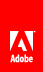// Ethnio survey code removed